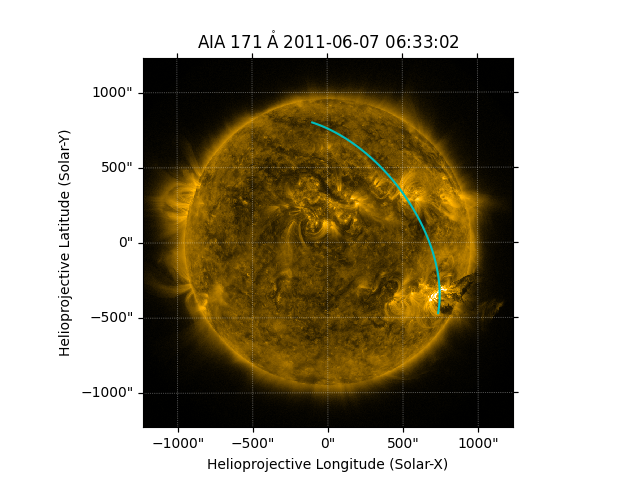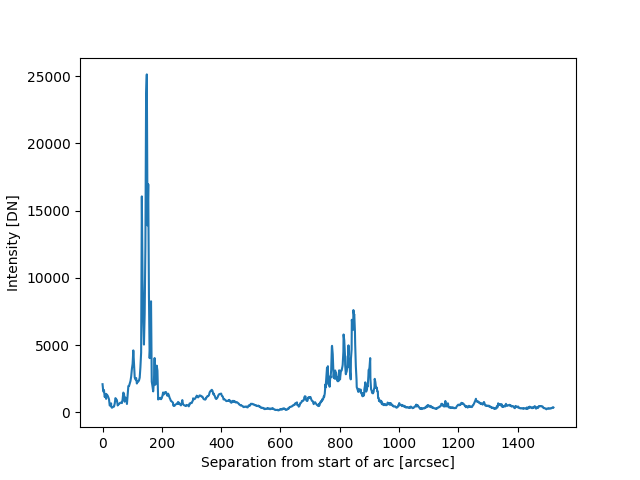# Drawing and using a Great Arc#

How to define and draw a great arc on an image of the Sun, and to extract intensity values along that arc.

import matplotlib.pyplot as plt

import astropy.units as u
from astropy.coordinates import SkyCoord
from astropy.visualization import quantity_support

import sunpy.map
from sunpy.coordinates.utils import GreatArc
from sunpy.data.sample import AIA_171_IMAGE

quantity_support()

<astropy.visualization.units.quantity_support.<locals>.MplQuantityConverter object at 0x7fb37e0abee0>


m = sunpy.map.Map(AIA_171_IMAGE)


Let’s define the start and end coordinates of the arc.

start = SkyCoord(735 * u.arcsec, -471 * u.arcsec, frame=m.coordinate_frame)
end = SkyCoord(-100 * u.arcsec, 800 * u.arcsec, frame=m.coordinate_frame)


Create the great arc between the start and end points.

great_arc = GreatArc(start, end)


Plot the great arc on the Sun.

fig = plt.figure()
m.plot(axes=ax, clip_interval=(1, 99.99)*u.percent)
ax.plot_coord(great_arc.coordinates(), color='c')[<matplotlib.lines.Line2D object at 0x7fb37f3a91e0>]


Now we can get the intensity along the great arc coordinates, along with the angular distance from the start of the arc

coords = great_arc.coordinates()
intensity_coords = sunpy.map.pixelate_coord_path(m, coords)
intensity = sunpy.map.sample_at_coords(m, intensity_coords)
separation = intensity_coords.separation(intensity_coords).to(u.arcsec)


Plot the intensity along the arc from the start to the end point.

fig, ax = plt.subplots()
ax.plot(separation, intensity)
ax.set_xlabel(f'Separation from start of arc [{separation.unit}]')
ax.set_ylabel(f'Intensity [{intensity.unit}]')

plt.show()Total running time of the script: (0 minutes 0.525 seconds)

Gallery generated by Sphinx-Gallery